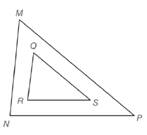Chapter 5.2, Problem 9E### Elementary Geometry for College St...

6th Edition
Daniel C. Alexander + 1 other
ISBN: 9781285195698

#### Solutions

Chapter
Section### Elementary Geometry for College St...

6th Edition
Daniel C. Alexander + 1 other
ISBN: 9781285195698
Textbook Problem
1 views

# Given: △ M N P ∼ △ Q R S , m ∠ M = 56 ∘ , m ∠ R = 82 ∘ , M N = 9 , Q R = 6 , R S = 7 , M P = 12 Find: a) m ∠ N c) N P b) m ∠ P d) Q STo determine

a)

To find:

mN.

Explanation

Definition used:

Similarity between Two polygons:

Two polygons are similar if and only if when all pairs of corresponding angles are congruent and all pairs of corresponding sides are proportional.

Given:

ΔMNPΔQRS,mM=56,mR=82,MN=9,QR=6,RS=7,MP=12

Calculation:

We have ΔMNPΔQRS

To determine

b)

To find:

mP.

To determine

c)

To find:

NP.

To determine

d)

To find:

QS.

### Still sussing out bartleby?

Check out a sample textbook solution.

See a sample solution

#### The Solution to Your Study Problems

Bartleby provides explanations to thousands of textbook problems written by our experts, many with advanced degrees!

Get Started